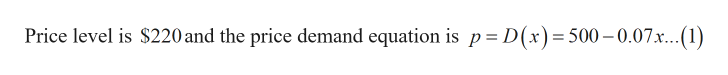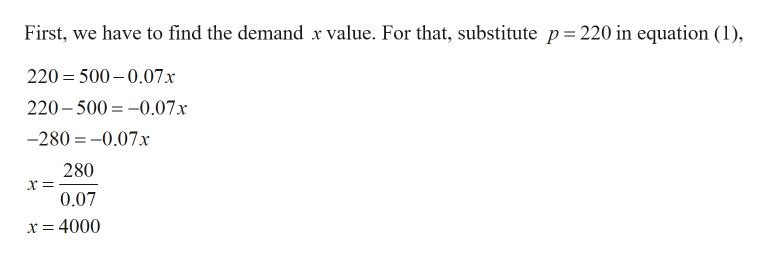# find the consumers surplus at a price level of \$220 for the price-demand equation p=D(x)=500-0.07x

Question
8 views

find the consumers surplus at a price level of \$220 for the price-demand equation p=D(x)=500-0.07x

check_circle

Step 1

Given:help_outlineImage TranscriptionclosePrice level is \$220 and the price demand equation is p = D(x)= 500-0.07x... (1) fullscreen
Step 2

Calculation:

Step 3help_outlineImage TranscriptioncloseFirst, we have to find the demand x value. For that, substitute p 220 in equation (1) 220 500-0.07x 220-500 0.07x -280-0.07x 280 0.07 x = 4000 fullscreen

### Want to see the full answer?

See Solution

#### Want to see this answer and more?

Solutions are written by subject experts who are available 24/7. Questions are typically answered within 1 hour.*

See Solution
*Response times may vary by subject and question.
Tagged in

### Other